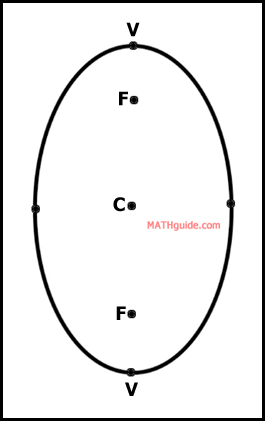Ellipse: Find an Equation Given Vertices & Foci
View the Lesson | MATHguide homepage Updated July 7th, 2021

Status: Read the following problem and respond accordingly. Waiting. . .

Determine the equation of an ellipse with the given information.

The vertices and foci are located at:

V(6,-8), V(6,6),
F(6,-4), F(6,2).

 (x - + )2 (y - + )2+= 1